Next: Colored Noise Up: APC591 Tutorial 7: A Previous: A Standard Wiener Process

# White Noise

White noise
is the formal derivative of a Wiener process(this is a formal derivative because
has probability one of being nondifferentiable). White noise has the properties

•
,
•.

For example, consider the stochastic differential equation
(1)

Here
represents the noise, and since
we interpret
as the r.m.s. noise strength. Multiplying equation (1) by
,
(2)

Let
Then
(3)

so
Finally, defining
and
,
(4)

This is easily programmed in Matlab, and for
gives a Wiener process such as that shown in Figure 1.

In general, for the stochastic differential equation
(5)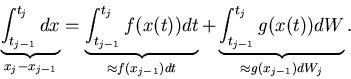Thus,
(6)

This is known as the Euler-Maruyama Method for solving stochastic differential equations.

For example, suppose that we want to solve the formal equation
(7)

or equivalently,
(8)

Note that this equation can be solved exactly, with
(9)

The following program em_simple.m is a slight modification of the program em.m from the article by Higham; it numerically solves equation (8) and compares to the exact solution.

%EM  Euler-Maruyama method on linear SDE
%
% SDE is  dX = lambda*X dt + mu*X dW,   X(0) = Xzero,
%      where lambda = 2, mu = 1 and Xzero = 1.
%
% Discretized Brownian path over [0,1] has dt = 2^(-8).
% Euler-Maruyama uses timestep dt.

randn('state',100)
lambda = 2                        % problem parameters
mu = 1;
Xzero = 1;
T = 1;
N = 2^8;
dt = 1/N;

dW = sqrt(dt)*randn(1,N);         % Brownian increments
W = cumsum(dW);                   % discretized Brownian path

Xtrue = Xzero*exp((lambda-0.5*mu^2)*([dt:dt:T])+mu*W);
plot([0:dt:T],[Xzero,Xtrue],'m-'), hold on

Xem = zeros(1,N);                 % preallocate for efficiency

Xem(1) = Xzero + dt*lambda*Xzero + mu*Xzero*dW(1);

for j=2:N
Xem(j) = Xem(j-1) + dt*lambda*Xem(j-1) + mu*Xem(j-1)*dW(j);
end

plot([0:dt:T],[Xzero,Xem],'b--*'), hold off
xlabel('t','FontSize',12)
ylabel('x','FontSize',16,'Rotation',0,'HorizontalAlignment','right')

emerr = abs(Xem(end)-Xtrue(end))


This produces Figure 2: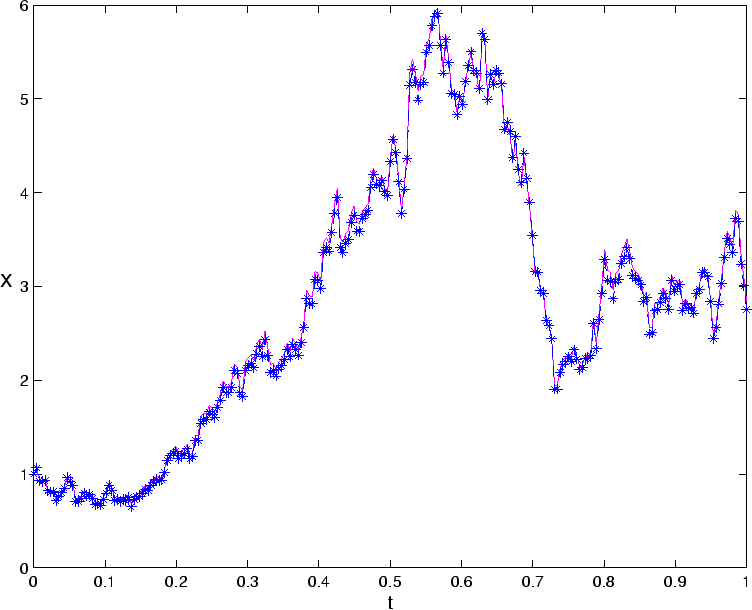Next: Colored Noise Up: APC591 Tutorial 7: A Previous: A Standard Wiener Process
Jeffrey M. Moehlis 2001-12-06